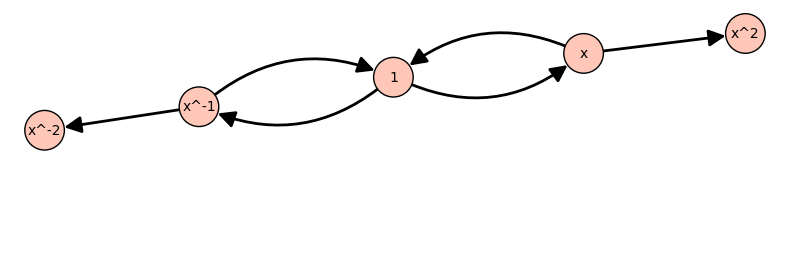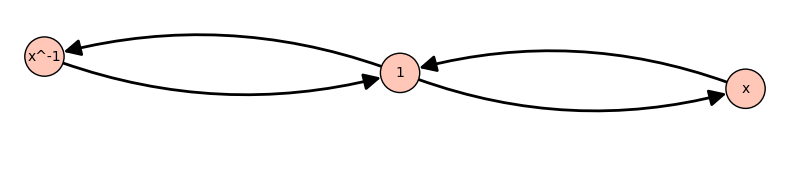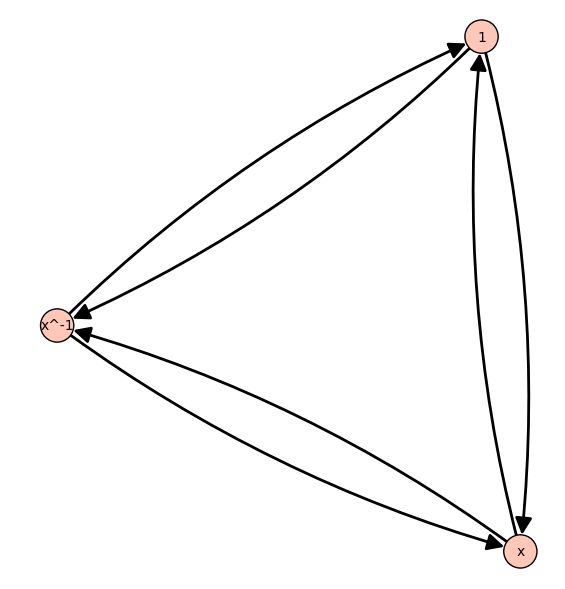# Cayley graphs for finitely presented groupsI am trying to produce Cayley graphs for finite groups defined using presentations, but I am getting strange results.

For example, the following should produce the Cayley graph for the cyclic group with 3 elements:

F.<x> = FreeGroup()
rel = [ x^3 ]
G = F/rel
C = G.cayley_graph()
C.plot()


but the graph produced has 5 vertices:If I tell Sage the list of elements like so:

F.<x> = FreeGroup()
rel = [ x^3 ]
G = F/rel
L = G.list()
C = G.cayley_graph(elements=L, generators=[x, x^-1])
C.plot()


then the number of vertices is correct, but there are no edges between $x$ and $x^{-1}(=x^2)$:Does anyone know how to fix this?

edit retag close merge delete

Sort by » oldest newest most voted

This has to do with rewriting systems for groups given by presentations.

When a group G is defined as a quotient of the free group by some relations, as in the question, elements in G are stored as words in the generators.

Deciding whether elements given by different words are equal in the group is undecidable in general, although of course for some groups it is decidable.

Technically speaking, a rewriting system is associated with the group presentation.

A rewriting system is called confluent if it provides a canonical representative for each group element.

The rewriting system associated with the group G as defined in the question is not confluent.

sage: F.<x> = FreeGroup('x')
sage: G = F / [x^3]

sage: r = G.rewriting_system()
sage: r
Rewriting system of Finitely presented group < x | x^3 >
with rules:
x^3    --->    1

sage: s = G.rewriting_system()
sage: s.make_confluent()
sage: s
Rewriting system of Finitely presented group < x | x^3 >
with rules:
x^-2    --->    x
x^2    --->    x^-1


To compare the initial rewriting system and the confluent one:

sage: r.is_confluent()
False
sage: s.is_confluent()
True


The initial one can only simplify positive multiples of 3 in the exponent, while the confluent one gives a unique form with exponents between -1 and 1.

sage: a = "  n    x^n   G(x^n)   r(x^n)   s(x^n)"
sage: b = "-------------------------------------"
sage: c = [(n, x^n, G(x^n), r.reduce(x^n), s.reduce(x^n)) for n in range(-5, 8)]
sage: print('\n'.join(['', a, b] + ["%3s %6s %8s %8s %8s" % t for t in c]))

n    x^n   G(x^n)   r(x^n)   s(x^n)
-------------------------------------
-5   x^-5     x^-5     x^-5        x
-4   x^-4     x^-4     x^-4     x^-1
-3   x^-3     x^-3     x^-3        1
-2   x^-2     x^-2     x^-2        x
-1   x^-1     x^-1     x^-1     x^-1
0      1        1        1        1
1      x        x        x        x
2    x^2      x^2      x^2     x^-1
3    x^3      x^3        1        1
4    x^4      x^4        x        x
5    x^5      x^5      x^2     x^-1
6    x^6      x^6        1        1
7    x^7      x^7        x        x


For some groups, attempting to make the rewriting system confluent will not terminate. This is why it is not done systematically.

The function my_cayley_graph below has an option to try and make the rewriting system confluent.

When this succeeds, this guarantees a correct Cayley graph.

def my_cayley_graph(self, side="right", simple=False, elements=None,
generators=None, connecting_set=None,
make_rewriting_system_confluent=False):
r"""
Return the Cayley graph for this finite semigroup.

INPUT:

- side -- "left", "right", or "twosided":
the side on which the generators act (default:"right")
- simple -- boolean (default:False):
if True, returns a simple graph (no loops, no labels,
no multiple edges)
- generators -- a list, tuple, or family of elements
of self (default: self.semigroup_generators())
- connecting_set -- alias for generators; deprecated
- elements -- a list (or iterable) of elements of self
- make_rewriting_system_confluent -- whether to attempt
to make self's rewriting system confluent to compute
the Cayley graph (warning: this might not terminate)

Adapted from the cayley_graph method of semigroups, see Sage source code at
https://github.com/sagemath/sage/blob/9.8/src/sage/categories/semigroups.py#L174

OUTPUT:

- :class:DiGraph

EXAMPLES:

We illustrate the make_rewriting_system_confluent option::

sage: F.<x> = FreeGroup('x')
sage: G = F / [x^3]
sage: C = my_cayley_graph(G, make_rewriting_system_confluent=True)
sage: C.vertices(sort=False), C.edges(sort=False)
([1, x, x^-1],
[(1, x, 0), (1, x^-1, 1), (x, 1, 1), (x, x^-1, 0), (x^-1, 1, 0), (x^-1, x, 1)])

AUTHORS:

- Bobby Moretti (2007-08-10)
- Robert Miller (2008-05-01): editing
- Nicolas M. Thiery (2008-12): extension to semigroups,
side, simple, and elements options, ...
- Samuel Lelièvre (2023-05-19): add option to make rewriting system confluent
"""
from sage.graphs.digraph import DiGraph
from sage.categories.monoids import Monoids
from sage.categories.groups import Groups
if side not in ["left", "right", "twosided"]:
raise ValueError("option 'side' must be 'left', 'right' or 'twosided'")
if elements is None:
assert self.is_finite(), "elements should be specified for infinite semigroups"
elements = self
else:
elements = set(elements)
if simple or self in Groups():
result = DiGraph()
else:
result = DiGraph(multiedges=True, loops=True)

if connecting_set is not None:
generators = connecting_set
if generators is None:
if self in Monoids and hasattr(self, "monoid_generators"):
generators = self.monoid_generators()
else:
generators = self.semigroup_generators()
if isinstance(generators, (list, tuple)):
generators = dict((self(g), self(g)) for g in generators)
left  = (side == "left"  or side == "twosided")
right = (side == "right" or side == "twosided")
k = self.rewriting_system()
if make_rewriting_system_confluent:
k.make_confluent()
r"""
Add an appropriate edge to result given the options.

Skips edges whose targets are not in elements.
"""
source = k.reduce(source)
target = k.reduce(target)
if (elements is not self and target not in elements):
return
if simple:
if source != target:
elif side == "twosided":
else:
for x in elements:
for i in generators.keys():
if left:
add_edge(x, generators[i] * x, i, "left" )
if right:
add_edge(x, x * generators[i], i, "right")
return result


Example.

sage: F.<x> = FreeGroup('x')
sage: G = F / [x^3]

sage: C = my_cayley_graph(G, make_rewriting_system_confluent=True)

sage: C.vertices(sort=False), C.edges(sort=False)
([1, x, x^-1],
[(1, x, 0), (1, x^-1, 1), (x, 1, 1), (x, x^-1, 0), (x^-1, 1, 0), (x^-1, x, 1)])

sage: C
Digraph on 3 vertices

sage: C.plot()
Launched png viewer for Graphics object consisting of 12 graphics primitivesmore